Return to site

# The Advanced Guide to GT-POST Features

## Updated for v2017

As engineers, we're trained to optimize systems that we use to make better

decisions and solve problems.

In this guide, we want to share with you some advanced features that we use to

optimize the functionality of GT-POST.

All of us use GT-POST to analyze our simulation results or create plots to tell a

story.

Our goal is to present to you at least one new way to analyze your

results or create better plots.

We will be updating this guide with every new version of GT-SUITE and when we

have new methods to share.

We would love your feedback on:

• How you used this guide.
• Any suggestions you have to make the guide better.
• Any advanced features that you can share with us to help us master the tool further.

Table of contents:

1. Contour Plots of Test Data
2. Uniquely Sized Multiple Plots on Common X-Axis
3. Tables RLT's
4. Using Constraints with Plots

Contour Plots of Test Data

How can this feature help?

You can use GT-POST to create contour plots of any set of three-dimensional data. It can replace the effort it takes to create contour plots in a plotting tool like Concerto or writing a script in tools like Matlab.

Here are examples of where you can use this functionality:

• You can create test data contours and compare it with the simulation data contours. By using the same plotting tool you will get a consistent look.
• You can create 3D data contours for analysis and to understand the trend of your results. You can even use these plots to spruce up your presentations.

Creating contour plots of 3D data.

It is easy to create a contour plot of simulation results within GT-POST by using the GT-Macros (for e.g. “Create Plots of RLTs”)

What you probably didn’t know is that using GT-POST you can create plots of any data, even if it is not a gdx file.

This application is not limited to just test data. You can create contour plots of an analysis that you conducted in Excel, or even non-engineering data. Essentially, it can be any three-dimensional data that you have.

Step 1: Build a blank contour plot

Start GT-POST and open a new GU file. You can even use a pre-existing GU file. Then, right click the desired group title (see figure below) and select Add Plot.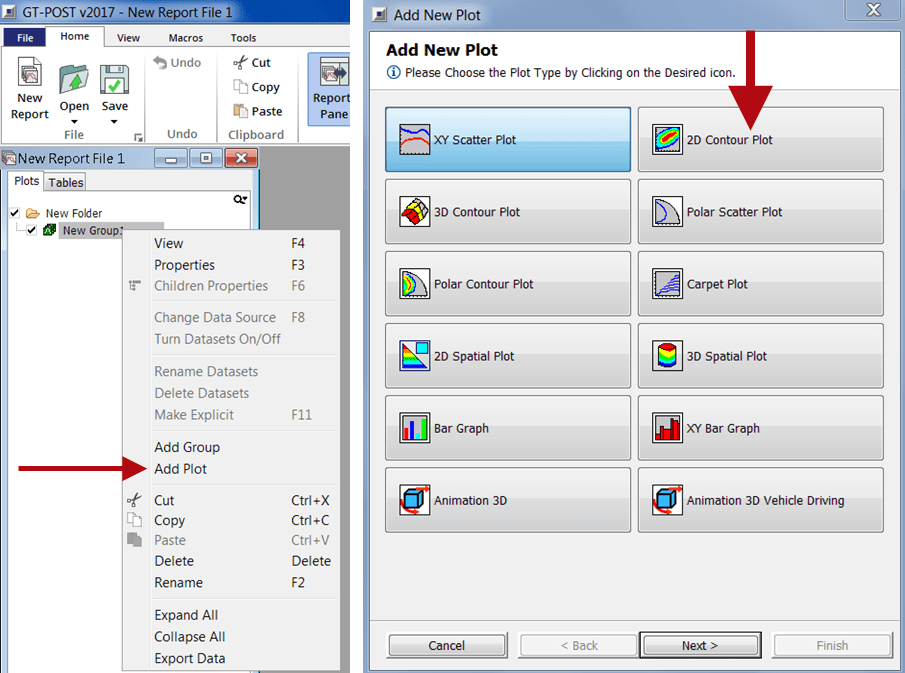Figure 1: Creating a template for the contour plot

You will see a new dialog box and you can create a plot with any of the available selections. For this example, we will select 2D Contour Plot.

In the next dialog boxes, we suggest leaving everything blank. This gives you the flexibility to change the X, Y and Z axis properties without having unit discrepancies or naming problems. This step makes life easier if you’re going to create multiple contour plots. When you hit Finish, you will see a plot in your GU file tree. This plot will have no data.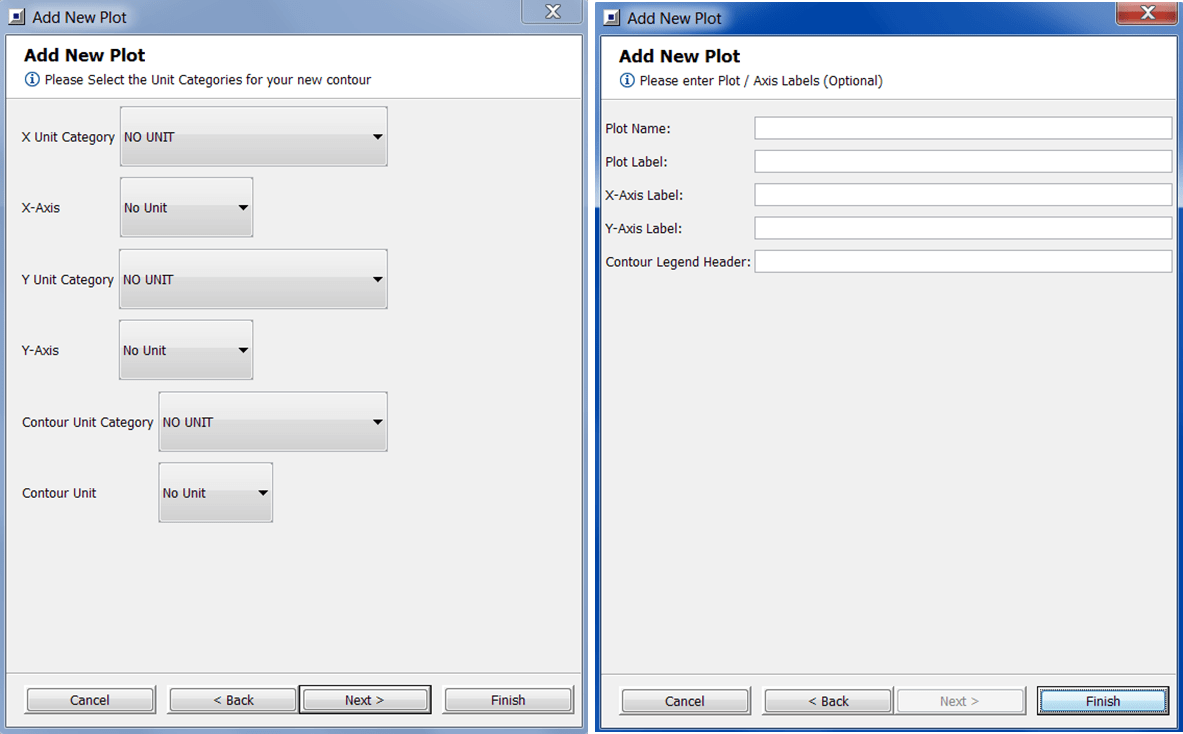Figure 2: Leave everything blank so that you have flexibility to change the X, Y, and Z axes.

Step 2A: Add data using the Explicit data source

Right click the new plot and select Add Data. You will see an Add Data Wizard open up. There are multiple choices of data types and multiple choices of data sources. In this guide, we will show you how you can add data using the Explicit and Excel data sources.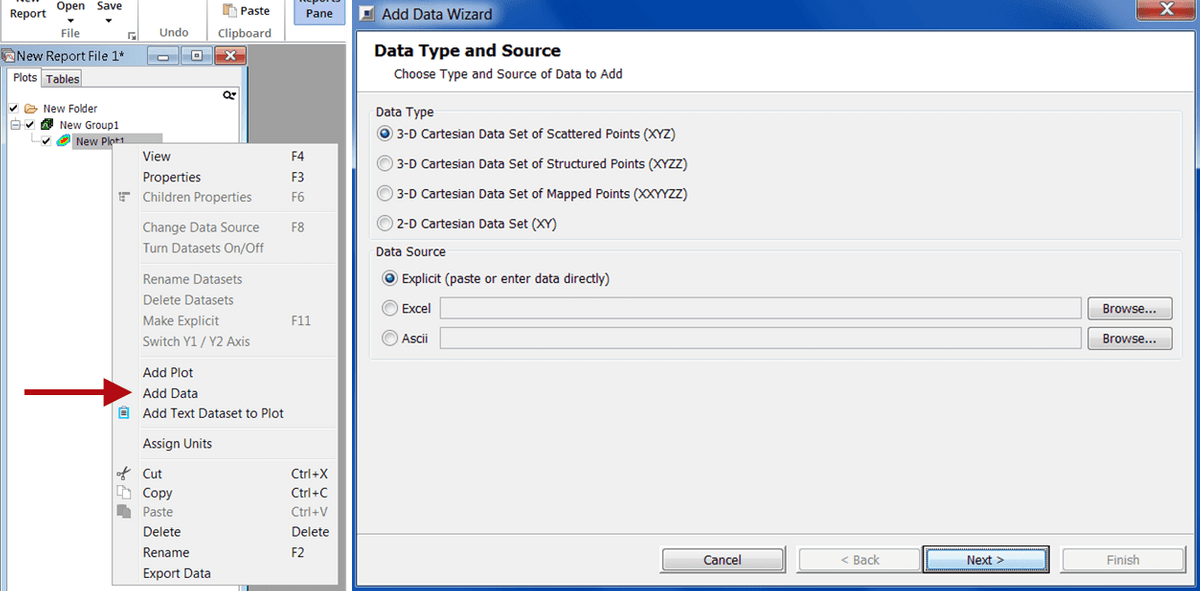Figure 3: Add data to the contour plot template you created.

Let’s begin by selecting Explicit as our data source and a data type of Scattered Points (XYZ). Scattered Points is the same as the XYZPoints reference table in GT-ISE.

The other common option is to select Structured Points (XYZZ), which allows you to add the data in the same way as you create a map. Structured Points is the same as the XYZMap reference table in GT-ISE.

When you hit Next a window will ask for the data name and unit for the X-Y-Z data. Since we selected No Unit while creating a blank plot, you will only be able to add a data name.

Once you hit next a data box opens up where you can manually feed in the data. Here’s how the data box will look like when you use either the Scattered Points or the Structured Points data type.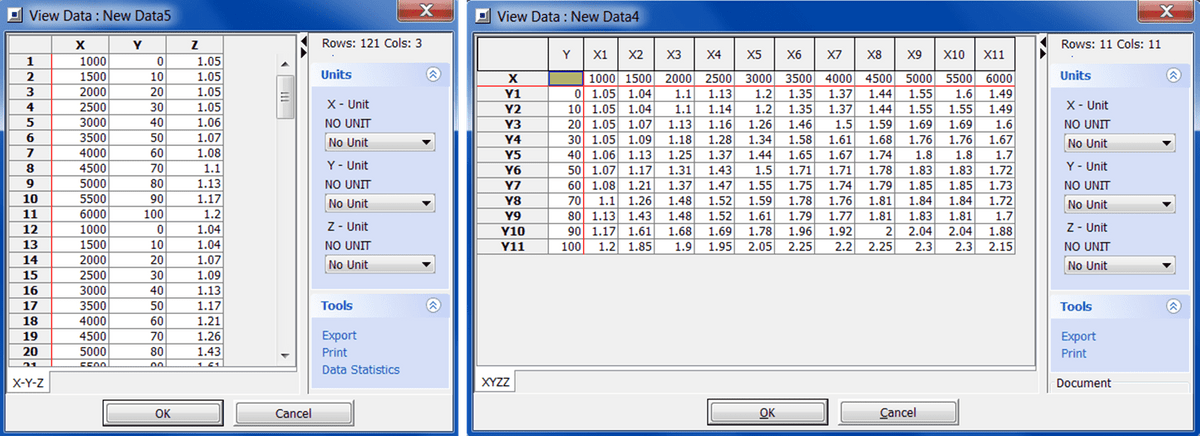Figure 4: Left - Adding data using Scattered Points. Right: Adding data using Structured Points.

When you hit OK a dataset is created. You can edit the plot properties by using the shortcut F3. Here you can add the details such as plot name, X-Y-Z axis labels and units.Figure 5: A contour plot created with a three-dimensional dataset.

Step 2B: Add data using the Excel data source

To add data using the Excel data source feature, similar to the previous step, right click on a plot and click Add Data. In the Add Data Wizard, select Excel as the Data Source and use the Browse button to locate your Excel file.

Hit Next and then select the data you want to import:

1. Select the columns you want to import. If your data is distributed in rows you can select the appropriate radio button in the middle of the file-and-selected data window.
2. After you select the columns, hit the right arrow button to transfer your selected data into the Selected Data window.
3. You can define the first row as the label and the second row as the unit depending on how your test data is set up.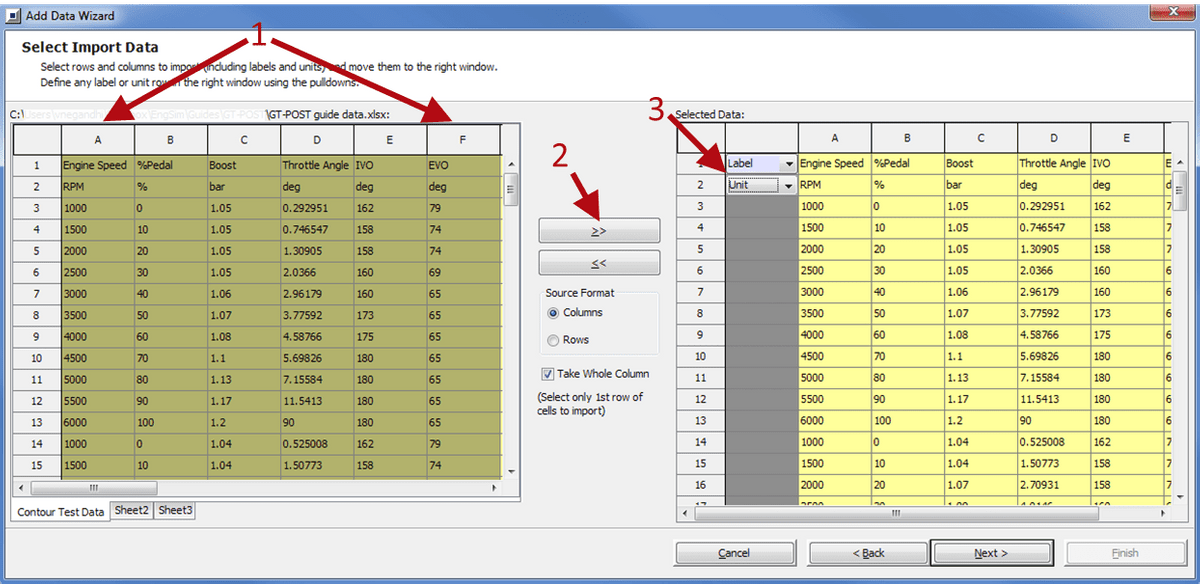Figure 6: Using Excel source to add the three-dimensional dataset.

When you hit Next and move on the next window, you will be able to define the data options. Which means that you can select which columns will be your X, Y and Z axis.

Since in our first steps of creating a blank plot we kept the units as No Unit you will not be able to define the units in this window. That is okay because you can write the appropriate units in the plot labels.

Before pressing Finish, you can decide whether you want to copy data from the Excel file (explicit link) or have the GU file link to your Excel file (implicit file pointer). If you link the data implicitly, you can use the data source feature to add the same data from another Excel sheet (a different test).

Hack: The implicit data linking is a powerful feature within GT-POST. This data handling method can save significant time in both simulation and experimental data comparisons and formatting. It is a key feature in making the argument for making GT-POST your all-purpose plotting tool.

Once you hit finish you will see that all the datasets are hosted under the same plot. You need to move each dataset under its own plot. To do that, you can either copy-paste this plot into multiple plots and delete all but one dataset. Or you can create another blank plot, make multiple iterations, and drag-drop each dataset into its own plot.

This step may sound confusing so to give some more clarity see Figure 7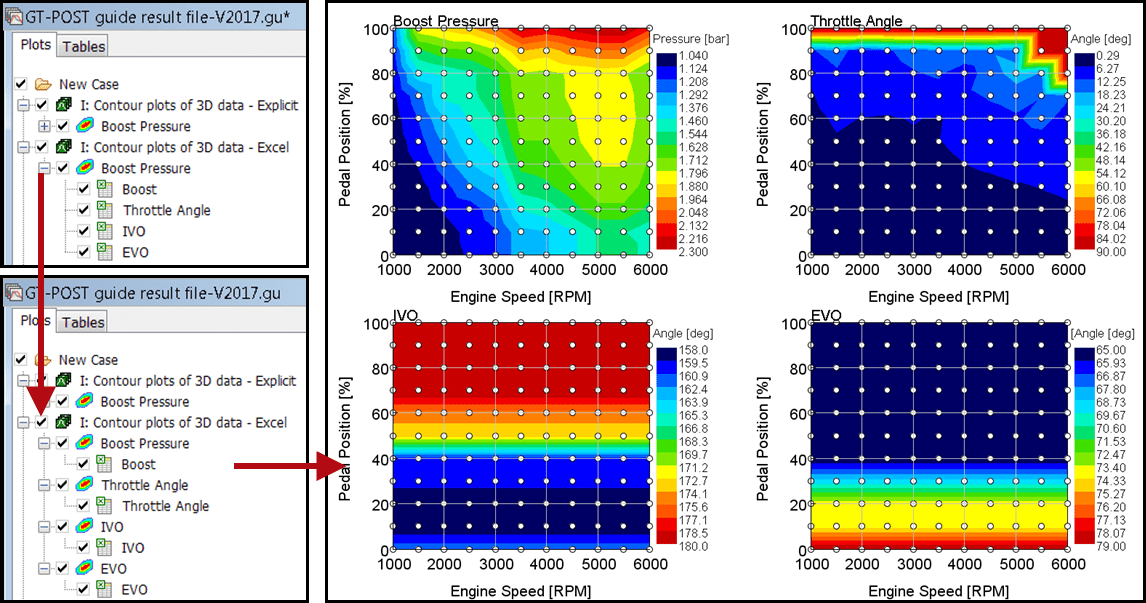Figure 7: Left - Transfer each dataset into its own plot. Right: Contour plots of three-dimensional data.

All steps to plot a contour plot of three-dimensional data is complete.

Hack: The white dots in the contour plots represent data points. To turn them on, you can go to the plot group - Children Properties (F6) - Data (hit next) - Deselect plots if you want to (hit Next) - Display - Turn on checkbox Show Symbol (at the bottom) - Hit Finish

Uniquely Sized Multiple Plots on Common X-Axis

How can this feature help?

Using XY plots of different sizes with a common X-axis, you can create better presentations.

If you want to show multiple properties on one slide and each have the same X-axis, then you can use this feature. You can highlight the important input to the system that is driving your results.

Here are some examples:

• In vehicle simulations, you can show how Target Vehicle Speed affects the vehicle performance.
• Figure 1 shows Engine Speed (output), Battery SOC (output), Distributor Mode (control), and Vehicle Speed (input) vs. Time (X-axis).
• It is a Time RLT result where each of the bottom two plots are occupying 20% of the plotting window and each of the top two plots occupies 30% of the window.
• In engine simulations, you can show the effect of changing Wastegate diameters for different engine speeds and its effect on engine properties.
• Figure 2 shows BMEP (output), BSFC (output), Wastegate Diameter (input) vs. Engine Speed (X-axis).
• These properties are Case RLT results where the BMEP, BSFC, and WG Dia occupy 45%, 30%, and 25% of the plot window respectively.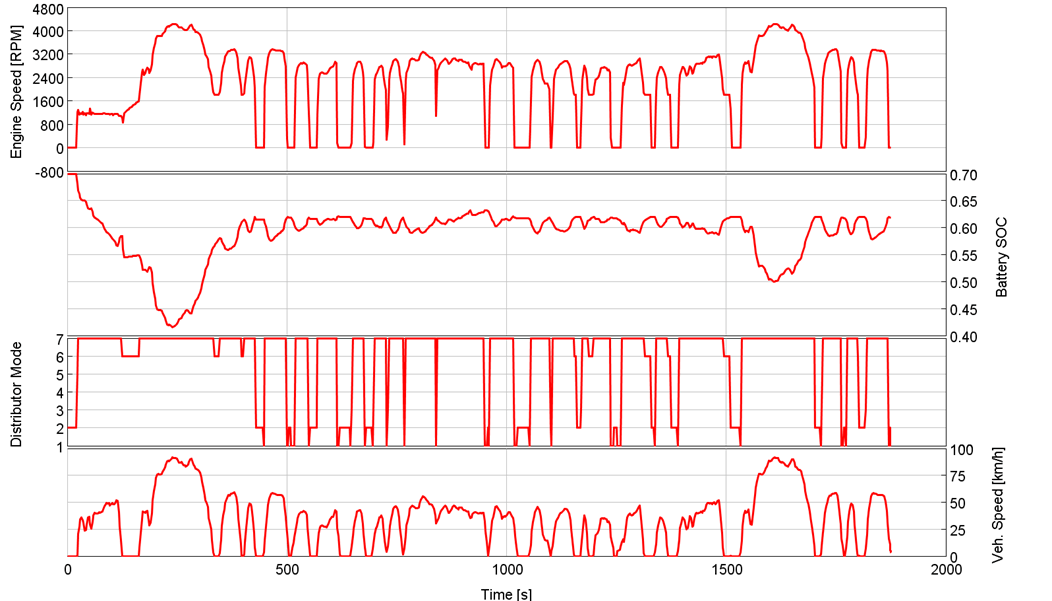Figure 1: Time RLT plots with common X-axis. Each plot size is uniquely defined by the user.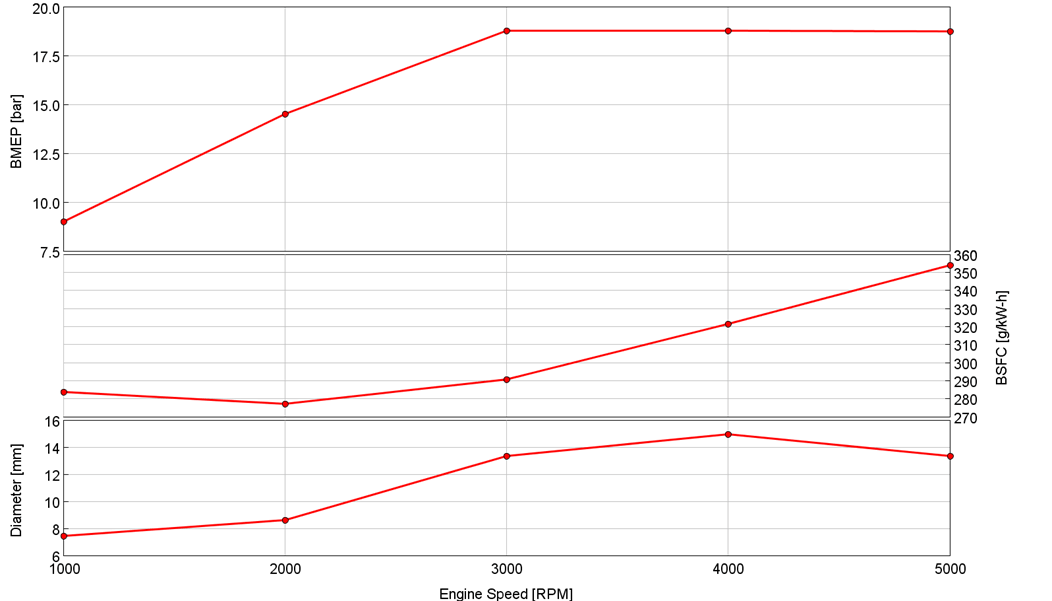Figure 2: Case RLT plots with common X-axis. Each plot size is uniquely defined by the user

Creating multiple plots on the same X-axis

In this post we’re going to show you:

• How to create a plot with a common X-axis
• How to size the different plots in the plot window

A - How to create a plot with a common X-axis

If you’re already familiar with how to create these common X-axis plots -- move on to part B - how to specify the size of each plot in the plot window.

For those of who haven’t created common X-axis plots before, here’s how the steps work.

Step 1 - Select the plot layout

Select the group under which your plots are nested. Right click on the group and go to Properties. You can even use the shortcut F3.

In the dialog box that opens up, on the top left you will see Main. In Main you change the Layout of plots to be 1x2, 1x3, or 1x4, depending on whether you want 2, 3 or 4 plots in the same plot window.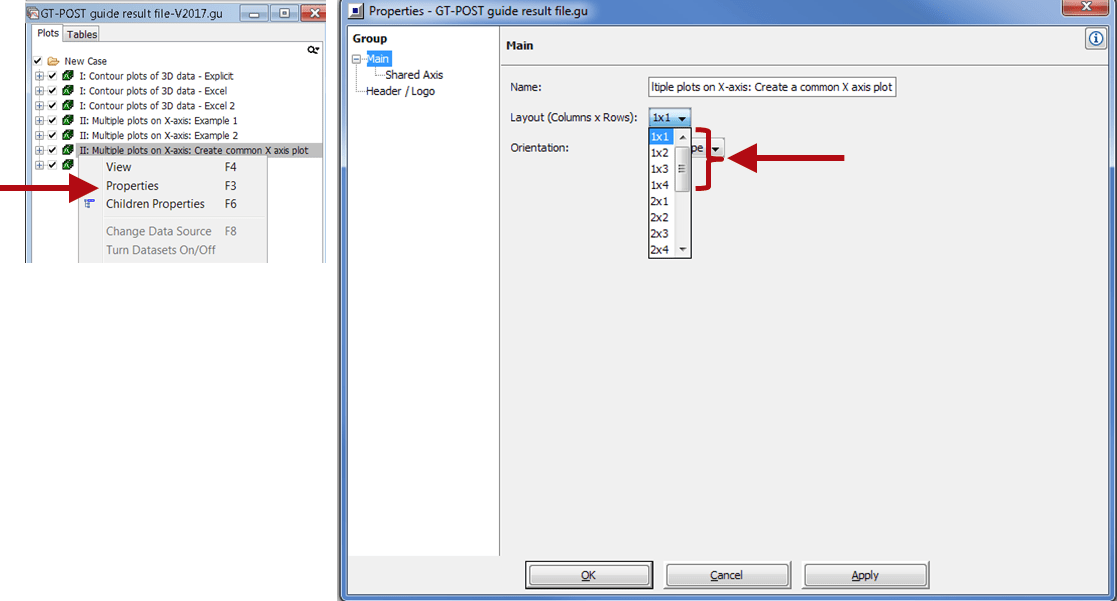Figure 3: Selecting the common X-axis plot layout

Step 2 – Define the shared axis properties

Under Main you will see Shared Axis, click on that. Now turn-on the first checkbox Share common axis (1 x N layout only).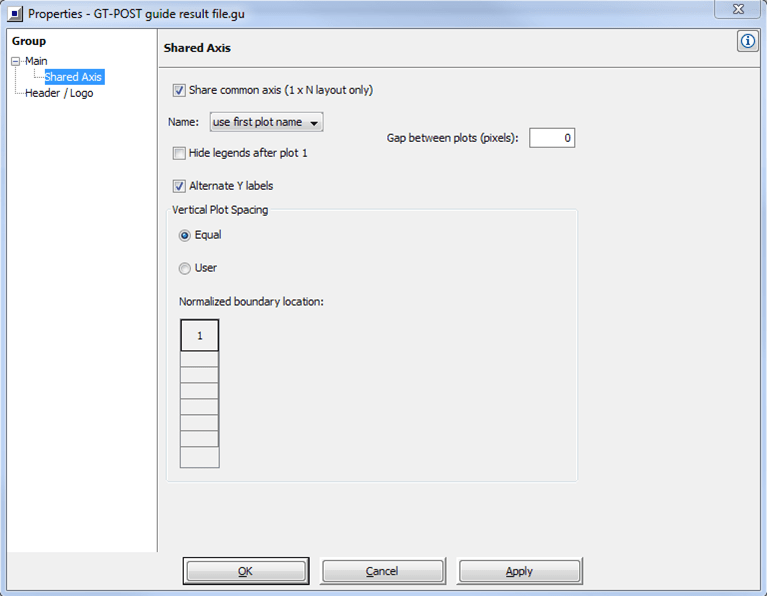Figure 4: Setting up the common X-axis plot

There are multiple parameters in this window:

• Name: You can choose the plot label to be -
• The name of the first plot
• The name of the group
• No name
• Gap between plots: If you desire there be white space between the plots, like the small spaces in Figure 1 and Figure 2. To put white space, you have to specify a number greater than zero.
• Check box to hide legends after plot 1. This option comes in handy if you have multiple datasets. For example, simulation and test. If this box is checked, it will hide the labels after plot 1.
• Vertical Plot Spacing: This option is the one where you specify the size of each plot.
• Equal: Means all plots will be sized equally.
• User: Will open up the Normalized boundary location matrix. You can use this matrix to size plots differently. We will show you how to do that in part B - how to specify the size of each plot in the plot window.

If you don’t change any of the other parameters in this Shared Axis window, you will create a plot window which uses the first plot name, has no gap between the plots, alternates the Y-axis labels, and are of equal size.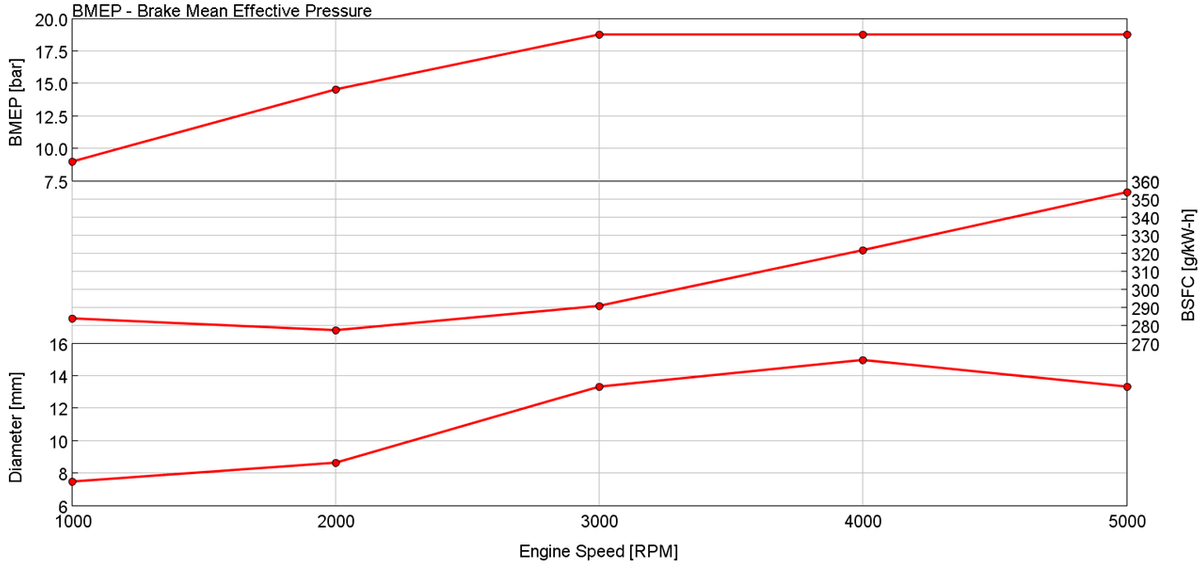Figure 5: A common X-axis plot with all properties under Shared Axis dialog box set to default

In the next step we will talk about how to change the size of the plots in the plot window using the User radio button.

B - How to specify the size of each plot in the plot window

To specify the size of each plot you need to the change the Vertical Spacing Option from Equal to User and fill in the Normalized Boundary Location Matrix.

How does the Normalized Boundary Location Matrix work?

At the start of this chapter, we showed you two examples of differently sized common X-axis plots.

• Figure 1 is a Time RLT result where the bottom two plots are occupying 20% of the plotting window each. The top two plots occupy 30% of the window each.
• Figure 2 is a Case RLT result where the BMEP, BSFC, and WG Dia occupy 45%, 30%, and 25% of the plot window respectively.

To create these plots we used the User option in the Normalized Boundary Location Matrix.

• Figure 1: The rows of the matrix were 0, 0.2, 0.4, 0.7, 1.
• Figure 2: The rows of the matrix were 0, 0.25, 0.55, 1.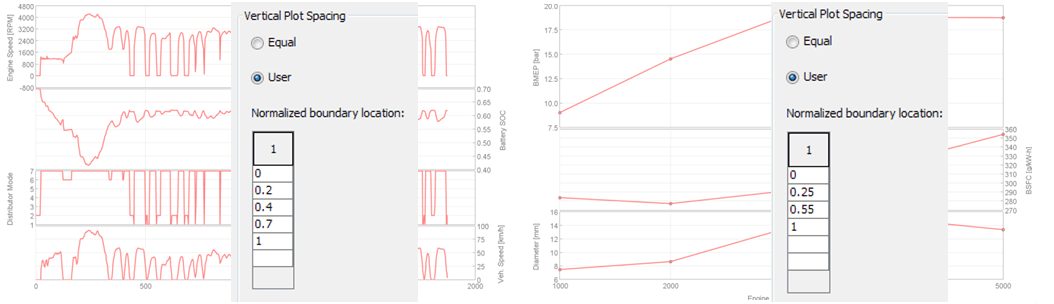Figure 6: Normalized boundary location matrix values for Example 1 and Example 2.

So what do the numbers in the location matrix mean?

It is the normalized location of the horizontal lines on the Y-axis of the plot window (Figure 7).

For the example with layout 1x4. Refer to figure 7:

• Start with 0 for plot 4 in the group tree. In his example it is Speed.
• The next number is 0.2: This is the normalized location on the Y-axis where plot 4 ends and plot 2 begins. Plot 4 is sized to be 20% of the entire plot window. Therefore we use 0 + 0.2 = 0.2
• The next number is 0.4: This is the normalized location on the Y-axis where plot 3 ends and plot 2 begins. Plot 3 is sized to be 20% of the entire plot window. Therefore we use 0.2 + 0.2 = 0.4
• The next number is 0.7: This is the normalized location on the Y-axis where plot 2 ends and plot 1 begins. Plot 3 is sized to be 20% of the entire plot window. Therefore we use 0.4 + 0.3 = 0.7
• End with 1 for plot 1 in the group tree. The last plot occupies 30% of the window because 0.7 + 0.3 = 1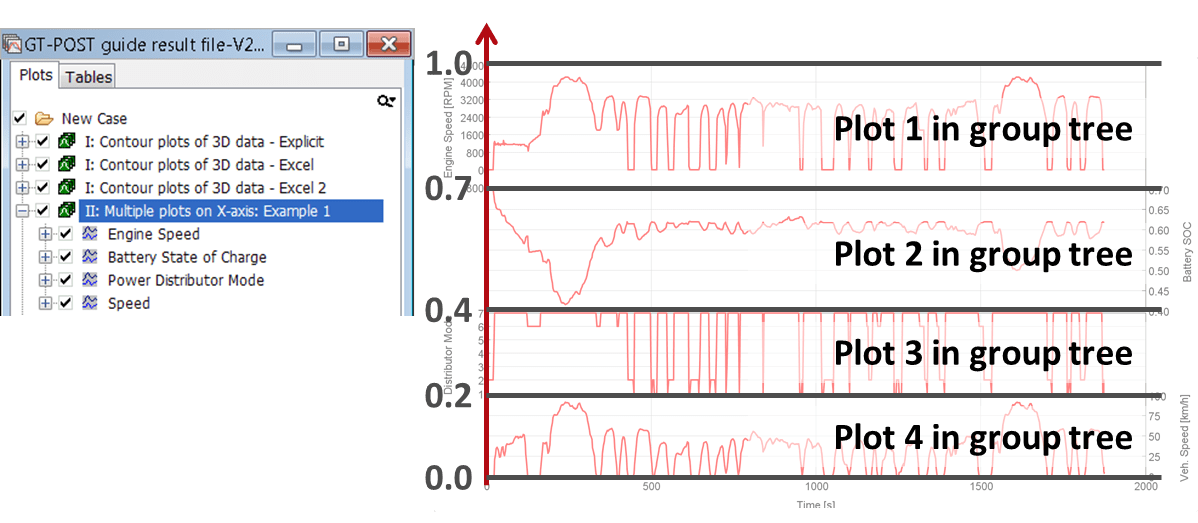Figure 7: How to define the numbers in the Normalized Boundary Location Matrix

In the examples, you will notice a system that GT wants us to follow.

The rules of this system are:

1. The boundary locations have to start from 0 and end at 1
2. The boundary locations have to be monotonically increasing, which means that the numbers should always be increasing from 0 to 1
3. In the boundary location matrix, the total number of rows will be one more than the total number of plots on the Y-axis. For example
• If you have a layout of 1x2, you need to fill 3 rows of the boundary location matrix.
• Continuing the example, if your layout is 1x3, you need to fill 4 rows, if 1x4, then 5 rows.

Now that you know the rules and meaning of the numbers in the boundary location matrix, you can create multiple unequal sized plots on the same X-axis using any of these three layouts (1x2, 1x3, and 1x4).

Tables of RLT's

How can this feature help?

We as engineers use post-processing tools either to analyze data or present the results. There are instances in analyzing results where we can’t make sense of the plots. There are also instances where we just can’t present the results in the right way.

In situations where the native plots in GT-POST just don’t cut it, you can use the Tables of RLT's feature to export raw data. Then you can use the raw data to debug issues or use another plotting tool to present the data.

Here are two examples how we use the Tables of RLT’s feature:

1. For plotting reasons.
• Figure 1: At times when we want to create a plot that GT-POST can’t, we use the Tables of RLT's feature. We then copy paste the data and build our own plots.
• Figure 2: When we want to plot the data using our company theme colors.
2. When we’re running a lot of cases and a problem occurs in some cases but not all, it is difficult to understand the results using graphs - because you have to remember the cases where you noticed the error. By creating Tables of RLT's and exporting the data to Excel, you can use the Conditional Formatting feature to highlight the cases where you see the problem.
• Figure 3 shows an example of highlighting cases where we don’t achieve a target CA50 of 8 crank angle degrees. For us, that would mean that the controller was not able to eliminate the error and we should calibrate our gains.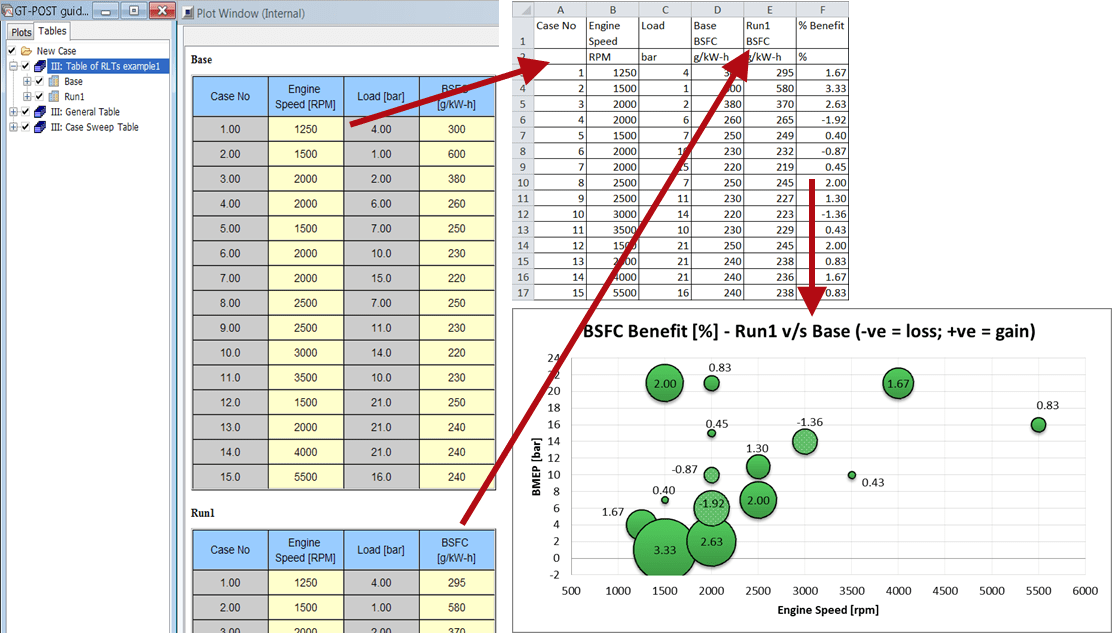Figure 1: Using the Tables of RLT feature to export raw data and create custom charts that are not possible with GT-POST.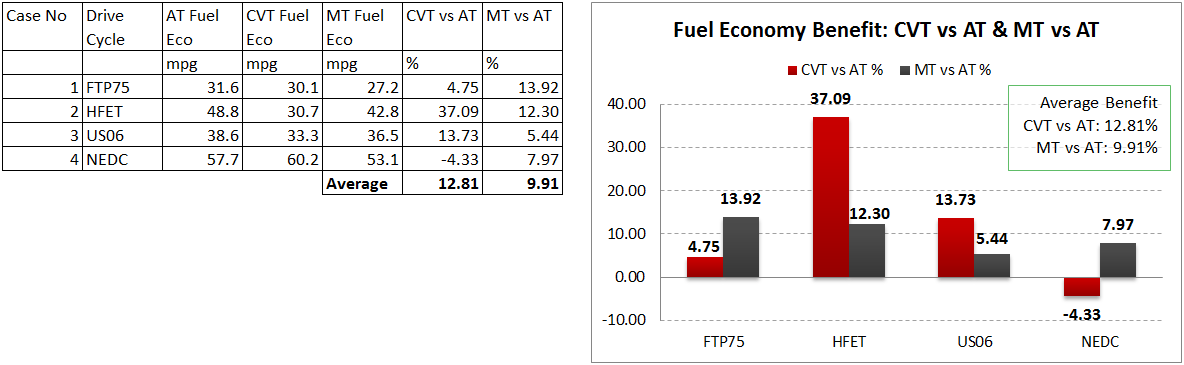Figure 2: Generating raw data using Tables of RLT’s and exporting data in Excel to create plots with the Company Theme colors.Figure 3: Generating raw data using Tables of RLT’s and exporting data in Excel to use the Conditional Formatting feature and debug results

Creating Tables of RLT's

By using this feature you can create either General Tables of RLT's or Case Sweep Tables of RLT's.

Both the types of tables report the Case RLTs of the simulation. You can plot Case RLTs of both periodic and continuous simulations. There are, however, two differences between General and Case Sweep Tables.

General Table of RLT's

• You have the ability to choose the cases for which the data will be generated
• In the table tree, each dataset will have multiple RLTs nested under each case

Case Sweep Table of RLT's

• By default, it will generate data for all cases
• In the table tree, each RLT you select will be its own dataset

The last point in the above table is confusing. We will explain that point using figures in the following sections.

A - How to create General Tables of Time RLT's

For this guide, we are going to use the GT-SUITE example Dynamic-AT. With the gdx for Dynamic-AT selected, hit the Create a Tables of RLT's macro button.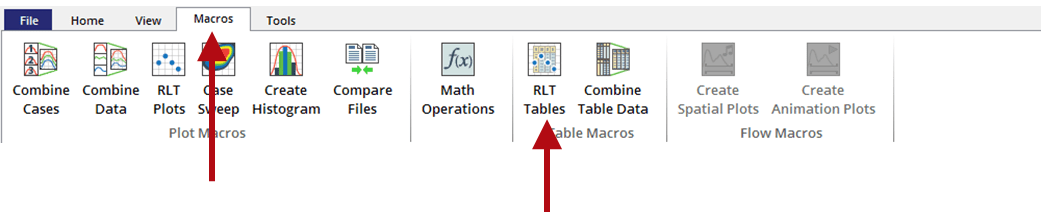Figure 4: The Tables of RLT’s Macro

A new dialog box will open up. For the table type we will choose General and we will turn on the Transpose checkbox. Next, we will populate the RLT table by selecting the RLTs from the the Tree. In the Selected RLTs section we can change the label for each.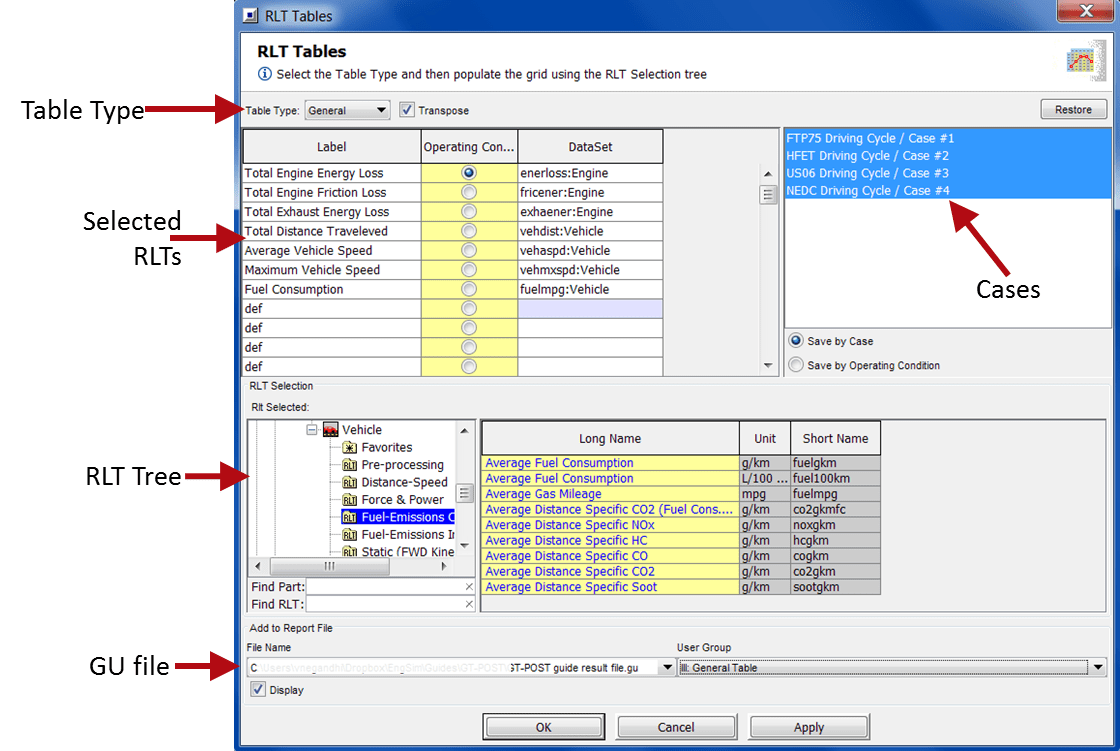Figure 5: RLT Tables dialog box and its different properties

The next step is to select the cases. In this example, we will select all the cases. The ability to select the cases which are of interest to you is the advantage of using the General type of Tables.

Finally, we will define the File Name and the User Group under which the table will be posted.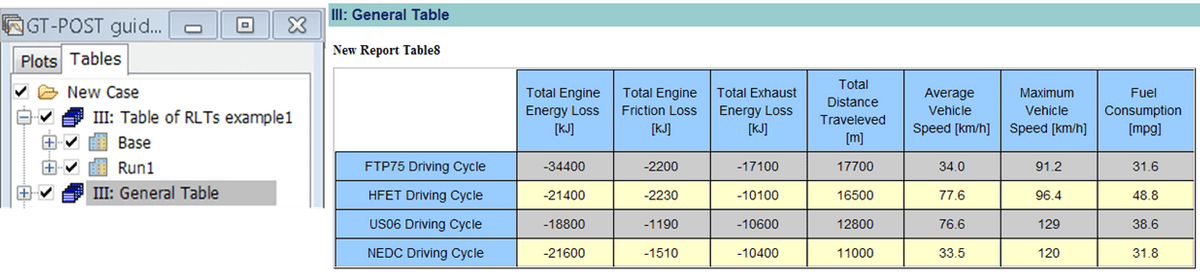Figure 6: Creating a Tables of RLT’s using the General type.

Hack: While selecting the File Name in the RLT Tables dialog box, you can select Export To Text File if you want to export the data instead of creating a table.

B - How to create a Case Sweep Tables of RLT’s

Select the gdx file Dynamic-AT and select the Create a Table of RLT's macro button. When the RLT Table dialog box opens up, select Case Sweep from the drop down menu. Next, we will populate the RLT table by selecting the RLTs from the RLT Tree. In the Selected RLTs section we can change the label for each.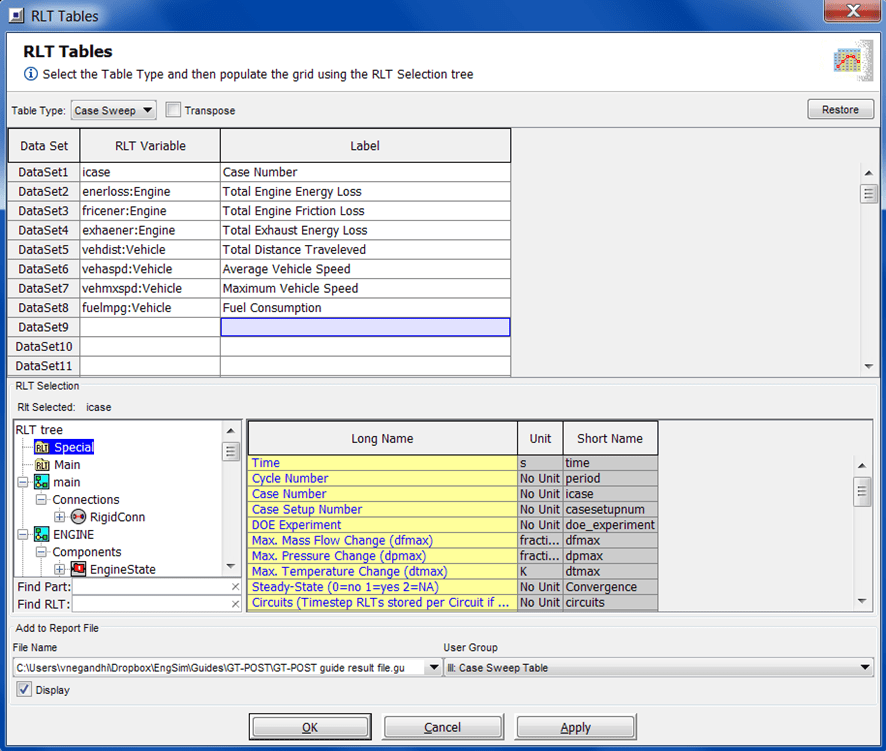Figure 7: RLT Tables dialog box for Case Sweep type of tables

In the Case Sweep Table type, all cases are selected by default.

Finally, we will define the File Name and the User Group under which the table will be posted.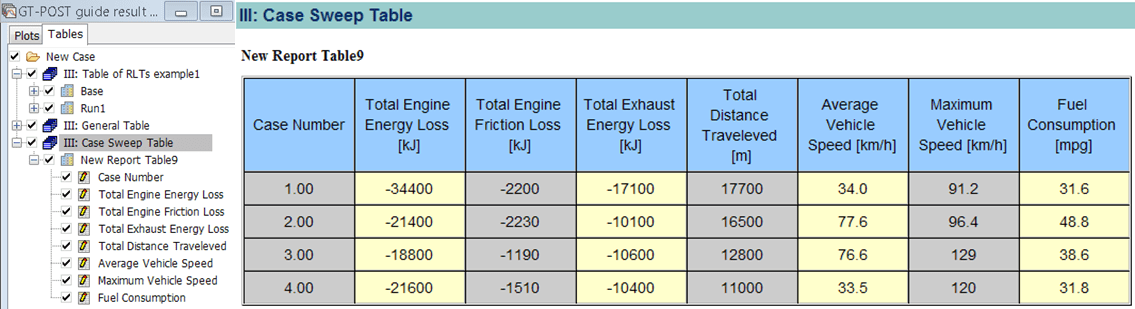Figure 8: Creating a Tables of RLT’s using the Case Sweep type.

Hack: Under this type of table you can double click the data set (case number for example) and you can specify the Display Decimal Digits to set how many digits to display after the decimal point.

At the start of the guide, we discussed the differences between the General and Case Sweep tables. The second point of the comparison was a bit confusing.

General Table of RLT's

• You have the ability to choose the cases for which the data will be generated
• In the table tree, each dataset will have multiple RLTs nested under each case

Case Sweep Table of RLT's

• By default, it will generate data for all cases
• In the table tree, each RLT you select will be its own dataset

We want to give you more clarity about how the datasets appear differently using Figure 9.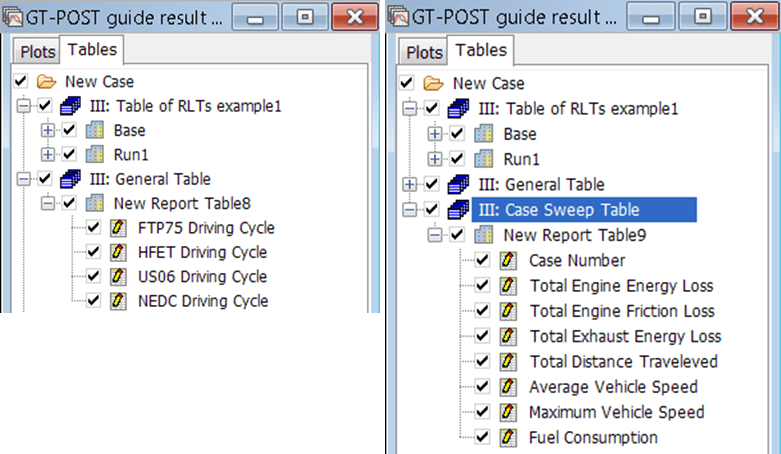Figure 9: Left - General type, the data sets are each case and all the RLTs are reported under each case.
Right - Case Sweep type, a unique data
set is created for each RLT and all case values are reported in each data set.

Using Constraints with Plots

How can this feature help?

Imagine yourself in a meeting where you’re presenting your results to a group of engineers.

You reach the slide with the most important result, share your observations, and someone asks, “How does [insert any property] look like for this result?” You might have that property plotted in the backup slides and you show it to your team.

Now, someone points out a problem, the result you’re showing is not valid because the property is outside the constraint it is designed for or the property has undesired levels.

For example, you present a result where at low speed you’re achieving a high torque. When someone asks about knock measurements you realize that the engine was knocking and now your results are invalid.

To avoid such situations and to get a better understanding of your results, you can add constraints to your plots.

Creating Plots using Constraints

To show how to use constraints to clean up your results, we are going to use the GT-SUITE example DOE_Pareto_Opt.

In this example, a 200-design-DOE is simulated. The Intake Cam Timing, Intake Runner Length, Exhaust Cam Timing, and the CA50 timing are the independent variables. The DOE is run for two operating conditions, 3000 RPM-WOT and 5000 RPM-WOT.

Adding constraints

To create a plot with constraints, open the gdx file for the model in GT-POST and select the macro button RLT Plots.Figure 1: Macro button for Create Plots of RLT’s

The Run RLT Macro dialog box opens up. In the dialog box select 2D Contour Plot and hit Next.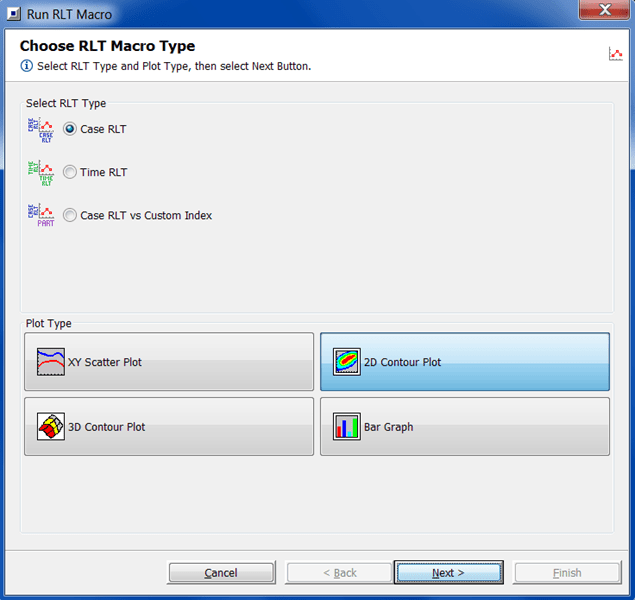Figure 2: Run RLT Macro dialog box

In Create Contour Plots, we’re going to use two independent variables as the X and Y-axis. In the RLT tree go to the Main folder and select “INTRUN-LENGTH” as X-Axis, and “thb50” as Y-axis. For the Z-axis, we will select “Brake Torque” from the Engine folder nested under RLT tree folder EngineCrankTrain.

As this model was ran at two conditions 3000 RPM and 5000 RPM, for clarity we want to look at the results only at one condition, 5000 RPM.

To generate the Brake Torque results only for 5000 RPM you will use the Constraints tab to create a constraint. From the RLT tree we will select “RPM” from the Main folder and set the Min and Max at 5000.

We also want to create another constraint for NOx emissions. Let’s assume that we have a limit of 4 g/kW-h of NOx.

You can create another constraint by hitting the “+” sign next to the 1st constraint. Then you can select the relationship of the 2nd constraint to the 1st. It can be either an “AND” or an “OR” relationship. In this case, we chose the AND relationship. Now, from the RLT tree - EngineCrankTrain - Engine - Emissions we choose “Brake Specific NOx - Cylinder Out,” set the Min as 0 and Max as 4 gm/kW-h.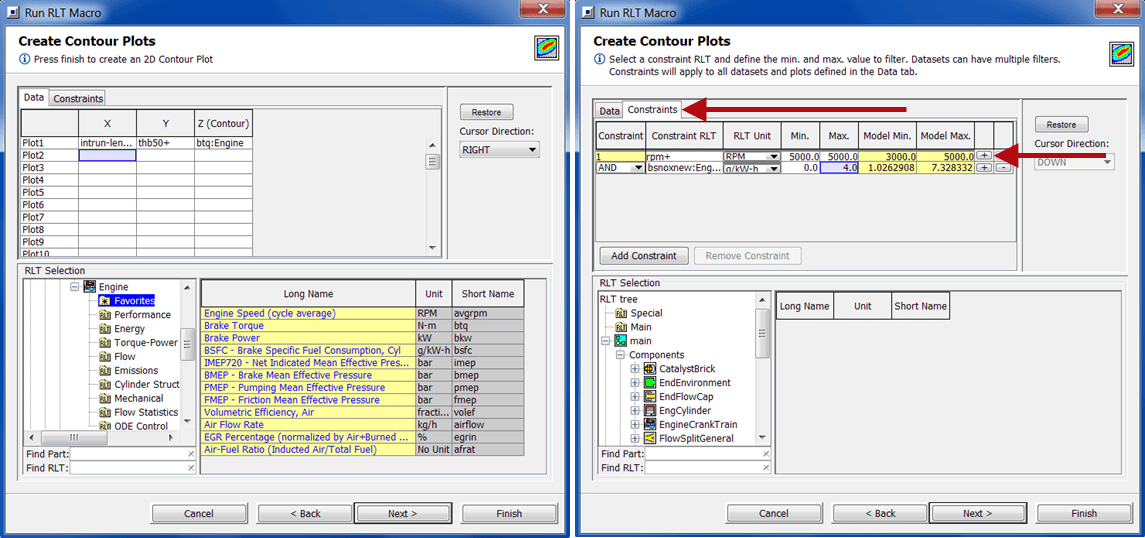Figure 3: Selecting the data to be plotted and setting the Engine RPM and NOx constraints

Once you hit Next, the Output Options dialog box will open up. Here you can chose how the plot label will be created, the GU file you want to add the plot to, and the group within the GU file. Once you hit Finish you will see the plot shown in Figure 4.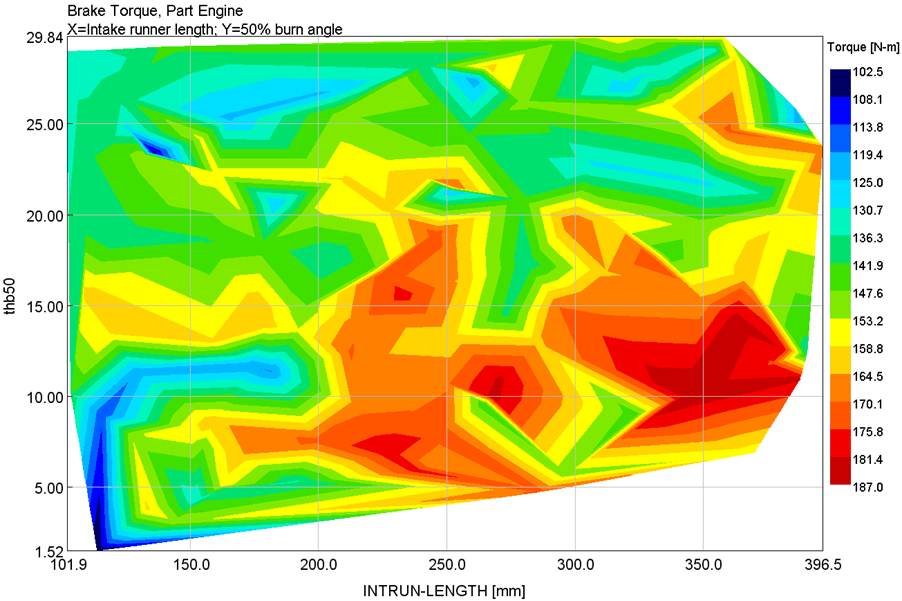Figure 4: Plot created for Brake Torque using the RPM and NOx constraints

Hack: Let’s say that you’ve created a plot and now you want to change a constraint. You can go the plot in the GU file, press the plus sign to locate the data and double click it. A new Properties/Data dialog box will open. In this dialog box on the left, under the XYZ data column you will see Source. When you click Source, you will see your constraints and you can change them, delete them and even add new constraints*.

* You cannot add a constraint after the plot is created, if there were “no” constraints set in the plot creation.

Adding constraints after the plot is created

If you want to add a constraint after the plot is created use the above Hack to locate the plot data and go to the Source tab in the Properties/Data dialog box.

In this dialog box you can change your existing constraints, delete constraints and add constraints.

We want to add a constraint to fix the lower limit of the Intake Cam Timing to 240 degrees. To add this constraint we will press the “+” sign next to the previous constraint, and select the constraint relationship “AND.” Then we will add the Constraint RLT “int-camtim+” and set the minimum to 240.Figure 5: Adding constraints after the plot is created

Here’s how the plots will look like before and after the intake constraint is added.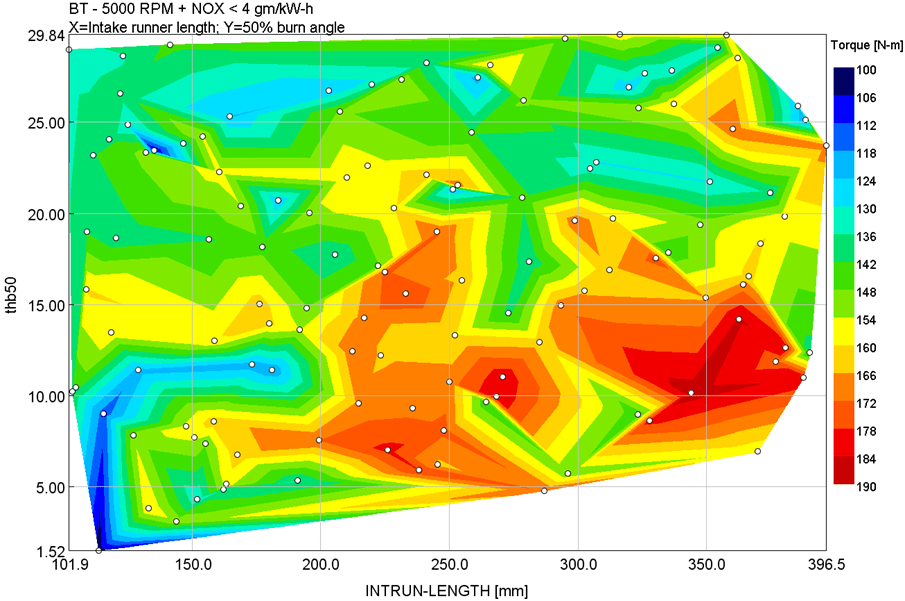Figure 6: Brake Torque with only the RPM and NOx constraints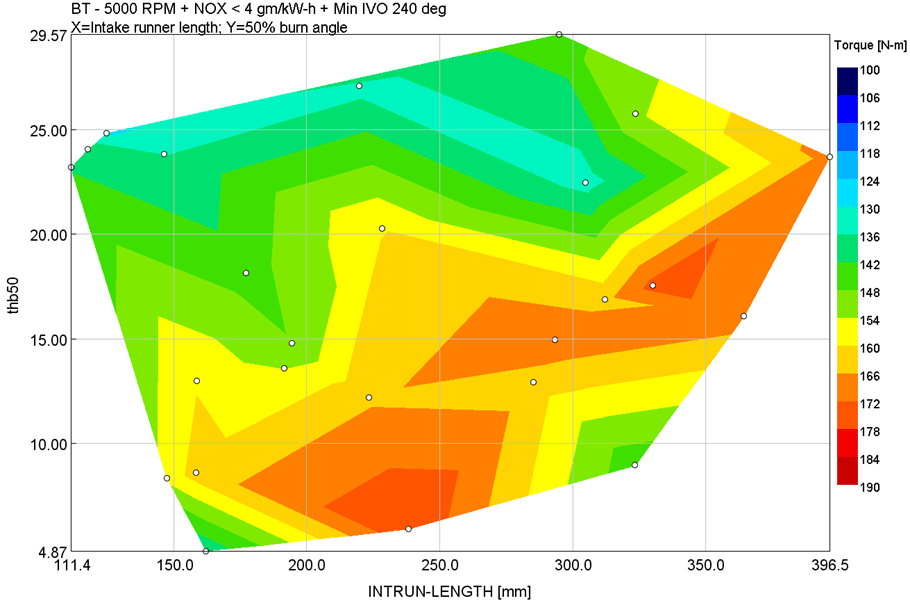Figure 7: Brake Torque with RPM, NOx, and Intake Cam Timing constraints

You can change the legend to be consistent in both the plots by right-clicking the parent plot group, and selecting Children properties. A dialog box will open up in which you can select the Plot radio button, hit Next and change the Contour Scale.

To add the white circles to represent the data points, go to Children properties, select the Data radio button, go to the Display tab and turn on the Show Symbols check box.

Comparing Figure 7 with Figure 6, you will notice how the viable area and the max brake torque reduces because of the added constraint. Even the data points defining the contour area reduces from Figure 6 to Figure 7.

That is how you create plots that include your system constraints, to ensure that your results are viable.

Thank you for reading

We would love to know if you found a tip that you didn't know and if you are willing to share a tip with us so that we as a community can get more expertise.

All Posts
×

Almost done…

We just sent you an email. Please click the link in the email to confirm your subscription!